# HSSlive: Plus One & Plus Two Notes & Solutions for Kerala State Board

## BSEB Class 9 Maths Chapter 14 Statistics Ex 14.3 Textbook Solutions PDF: Download Bihar Board STD 9th Maths Chapter 14 Statistics Ex 14.3 Book AnswersBSEB Class 9 Maths Chapter 14 Statistics Ex 14.3 Textbook Solutions PDF: Download Bihar Board STD 9th Maths Chapter 14 Statistics Ex 14.3 Book Answers

BSEB Class 9th Maths Chapter 14 Statistics Ex 14.3 Textbooks Solutions and answers for students are now available in pdf format. Bihar Board Class 9th Maths Chapter 14 Statistics Ex 14.3 Book answers and solutions are one of the most important study materials for any student. The Bihar Board Class 9th Maths Chapter 14 Statistics Ex 14.3 books are published by the Bihar Board Publishers. These Bihar Board Class 9th Maths Chapter 14 Statistics Ex 14.3 textbooks are prepared by a group of expert faculty members. Students can download these BSEB STD 9th Maths Chapter 14 Statistics Ex 14.3 book solutions pdf online from this page.

## Bihar Board Class 9th Maths Chapter 14 Statistics Ex 14.3 Books Solutions

 Board BSEB Materials Textbook Solutions/Guide Format DOC/PDF Class 9th Subject Maths Chapter 14 Statistics Ex 14.3 Chapters All Provider Hsslive

## How to download Bihar Board Class 9th Maths Chapter 14 Statistics Ex 14.3 Textbook Solutions Answers PDF Online?

2. Click on the Bihar Board Class 9th Maths Chapter 14 Statistics Ex 14.3 Answers.
3. Look for your Bihar Board STD 9th Maths Chapter 14 Statistics Ex 14.3 Textbooks PDF.
4. Now download or read the Bihar Board Class 9th Maths Chapter 14 Statistics Ex 14.3 Textbook Solutions for PDF Free.

Find below the list of all BSEB Class 9th Maths Chapter 14 Statistics Ex 14.3 Textbook Solutions for PDF’s for you to download and prepare for the upcoming exams:

## BSEB Bihar Board Class 9th Maths Solutions Chapter 14 Statistics Ex 14.3

Question 1.
A survey conducted by an organisation for the cause of illness and death among the women between the ages 15 – 44 (in years) worldwide, found the following figures (in %) :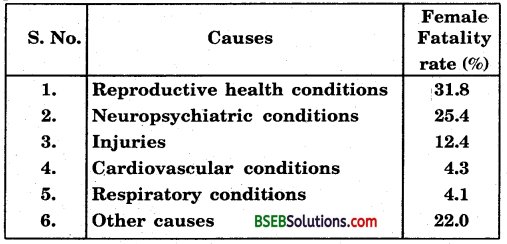(i) Represent the information given above graphically.
(ii) Which condition is the major cause of women’s ill health and death worldwide?
(iii) Try to find out, with the help of your teacher, any two factors which play a major role in the cause in (ii) above being the major cause.
Solution:
(i) The required graph is given on below:In the graph drawn causes of illness and death among women between the ages 15 – 44 (in years) worldwide is denoted on X-axis and female fatality rate (%) is denoted on the Y-axis.

(ii) The major cause of women’s ill health and death worldwide is reproductive health conditions.
(iii) Two other factors which play a major role in the cause in (ii) above are injuries and other causes.

Question 2.
The following data on the number of girls to the nearest ten per thousand boys in different sections of the society is given below: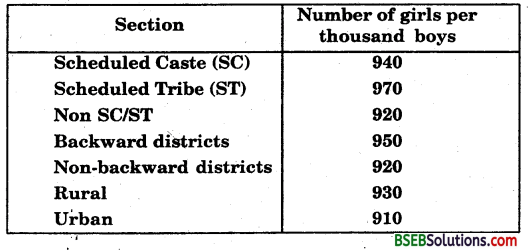(i) Represent the information above by a bar graph.
(ii) Write two conclusions you can arrive at from the graph, with justification.
Solution:
(i) The required graph is as under: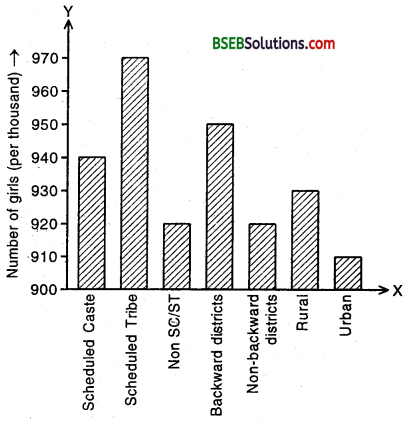In the graph drawn different sections of the society is denoted on the horizontal and the number of girls to the nearest ten per thousand boys is denoted on the vertical. Their intersection represent 900. Scale : 1 cm = 10 girls.

(ii) From, the graph we find that the number of girls to the nearest ten per thousand boys are maximum in scheduled tribes whereas they are minimum in urban.

Question 3.
Given below are the seats won by different political parties in the polling outcome of a state assembly elections:(i) Draw a bar graph to represent the polling results.
(ii) Which political party won the maximum number of seats ?
Solution:
In the graph drawn political party is denoted on the X-axis and the number of seat won on the Y-axis.
Scale : 1 cm = 10 seats.
The required graph is as follows :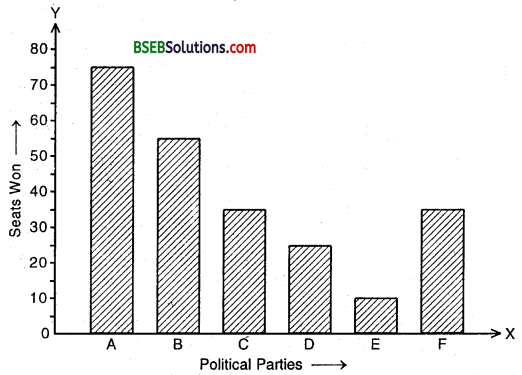(ii) Party A won the maximum number of seats.

Question 4.
The length of 40 leaves of a plant are measured correct to one millimetre, and the obtained data is represented in the table (given on the next page):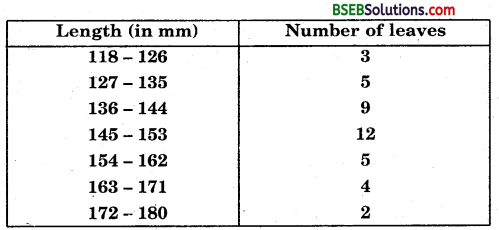(i) Draw a histogram to represent the given data.
(ii) Is there any other suitable graphical representation for the same data?
(iii) Is it correct to conclude that maximum number of leaves are 153 mm long? Why?
Solution:
(i) The given frequency distribution is not continuous. So, we shall first convert it into a continuous frequency distribution.

The difference between the lower limit of a class and the upper limit of the preceding class is 1. i.e., h = 1. To convert the given frequency distribution into a continuous frequency distribution, we subtract ℎ2 = 12 = 0.5 from each of lower limit and add 0.5 to each upper limit. The distribution so obtained is given as under:The histogram of the above frequency distribution is shown as under: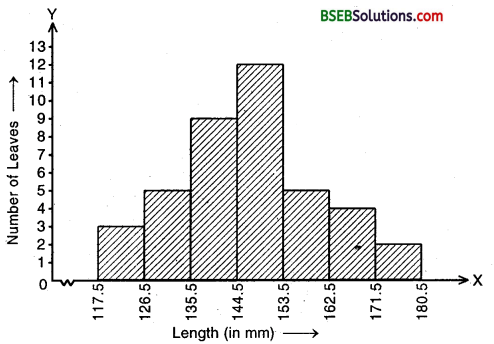(ii) Frequency polygon is another method of representing frequency distribution graphically.
(iii) Yes. as in the class interval 145 – 153, the lengths of leaves is maximum.

Question 5.
The following table gives the life times of 400 neon lamps: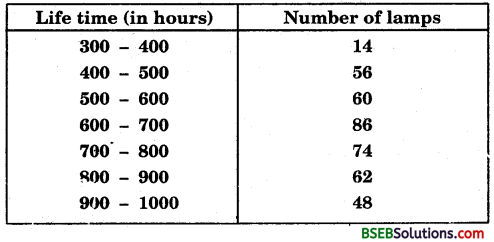(i) Represent the given information with the help of a histogram.
(ii) How many lamps have a life time of more than 700 hours ?
Solution:
(i) The histogram of the given frequency distribution is shown as under: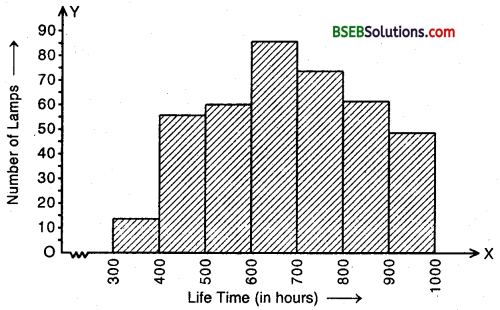(ii) Number of lamps having life more than 700 hours = 74 + 62 + 48 = 184.

Question 6.
The following two table gives the distribution of students of two sections according to the marks obtained by them :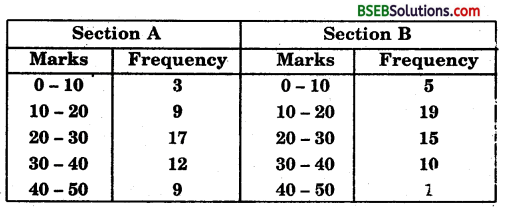Represent the marks of the students of both the sections on the same graph by two frequency polygons.
Solution:
First we obtain the class marks as given in the following table:We represent class marks on X-axis on a suitable scale and the frequencies onY-axis on a suitable scale.
To obtain the frequency polygon of section A, we plot the points (5, 3), (15, 9), (25, 17), (35, 12) and (45, 9) and join these points by line segments.
To obtain the frequency polygon of section B, we plot the points (5, 5), (15, 19), (25, 15), (35, 10) and (45, 1) on the same scale and join these points by dotted line segments.
The two frequency polygons are as shown below:Question 7.
The runs scored by two teams A and B on the first 60 balls in a cricket, match are given on the next pages.Represent the data of both the teams on the same graph by frequency polygons.
Solution:
First we obtain the class marks as given in the following table: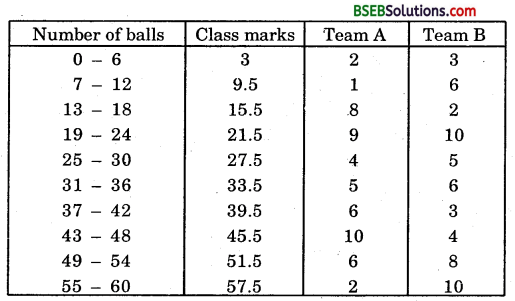We represent class marks on x-axis on a suitable scale and frequencies on y-axis on a suitable scale.
To obtain the frequency polygon of team A we plot the points (3, 2), (9.5, 1), (15.5, 8), (21.5, 9), (27.5, 4), (33.5, 5), (39.5, 6), (45.5, 10), (51.5, 6) and (57.5, 2) and join these points by the line segments.

To obtain the frequency polygon of team B, we plot the points (3, 3), (9.5, 6), (15.5, 2), (21.5, 10), (27.5, 5), (33.5, 6), (39.5, 3), (45.5, 4), (51.5, 8) and (57.5, 10).
The two frequency polygons are as shown below: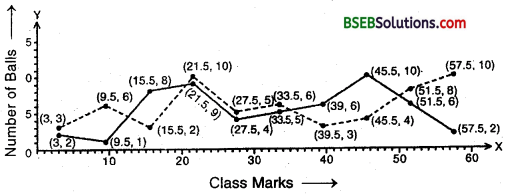Question 8.
A random survey of the number of children of various age groups playing in a park was found as follows:Draw a histogram to represent the data above.
Solution:
In the given frequency distribution, we see that the class-sizes are different. Hence, we calculate the adjusted frequency for each class by using the formula:In this problem, the minimum class-size = 2 – 1 = 1.
We thus obtain the following table of the adjusted frequency.We now represent the class intervals along the X-axis on a suitable scale and the corresponding adjusted frequencies on the Y-axis on a different suitable scale. Now, we draw rectangles with the class intervals as bases and the corresponding adjusted frequencies as the heights. The required histogram is as shown :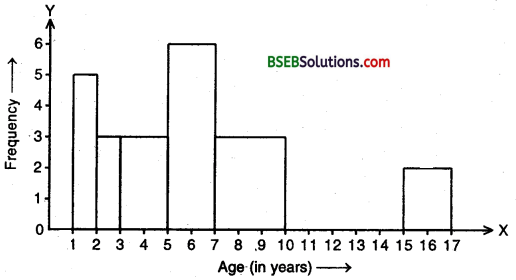Question 9.
100 surnames were randomly picked up from a local telephone directory and a frequency distribution of the number of letters in the English alphabets in the surnames was found as follows:(i) Draw a histogram to depict the given information.
(ii) Write the class interval in which the maximum number of surnames lie.
Solution:
(i) In the given frequency distribution, we see that the class-sizes are different. Hence, we calculate the adjusted frequency for each class by using the formula: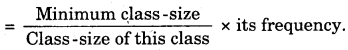In this problem, the minimum class-size = 6 – 4 = 2.
We thus obtain the following table of the adjusted frequency.We now represent the class intervals along the X-axis on a suitable scale and the corresponding adjusted frequencies on the Y-axis on a different suitable scale. Now, we draw rectangles with the class intervals as bases and the corresponding adjusted frequencies as the heights. The required histogram is as shown.(ii) The maximum of surnames lies in 6 – 8 class interval.

## Bihar Board Class 9th Maths Chapter 14 Statistics Ex 14.3 Textbooks for Exam Preparations

Bihar Board Class 9th Maths Chapter 14 Statistics Ex 14.3 Textbook Solutions can be of great help in your Bihar Board Class 9th Maths Chapter 14 Statistics Ex 14.3 exam preparation. The BSEB STD 9th Maths Chapter 14 Statistics Ex 14.3 Textbooks study material, used with the English medium textbooks, can help you complete the entire Class 9th Maths Chapter 14 Statistics Ex 14.3 Books State Board syllabus with maximum efficiency.

## FAQs Regarding Bihar Board Class 9th Maths Chapter 14 Statistics Ex 14.3 Textbook Solutions

#### Can we get a Bihar Board Book PDF for all Classes?

Yes you can get Bihar Board Text Book PDF for all classes using the links provided in the above article.

## Important Terms

Bihar Board Class 9th Maths Chapter 14 Statistics Ex 14.3, BSEB Class 9th Maths Chapter 14 Statistics Ex 14.3 Textbooks, Bihar Board Class 9th Maths Chapter 14 Statistics Ex 14.3, Bihar Board Class 9th Maths Chapter 14 Statistics Ex 14.3 Textbook solutions, BSEB Class 9th Maths Chapter 14 Statistics Ex 14.3 Textbooks Solutions, Bihar Board STD 9th Maths Chapter 14 Statistics Ex 14.3, BSEB STD 9th Maths Chapter 14 Statistics Ex 14.3 Textbooks, Bihar Board STD 9th Maths Chapter 14 Statistics Ex 14.3, Bihar Board STD 9th Maths Chapter 14 Statistics Ex 14.3 Textbook solutions, BSEB STD 9th Maths Chapter 14 Statistics Ex 14.3 Textbooks Solutions,
Share: